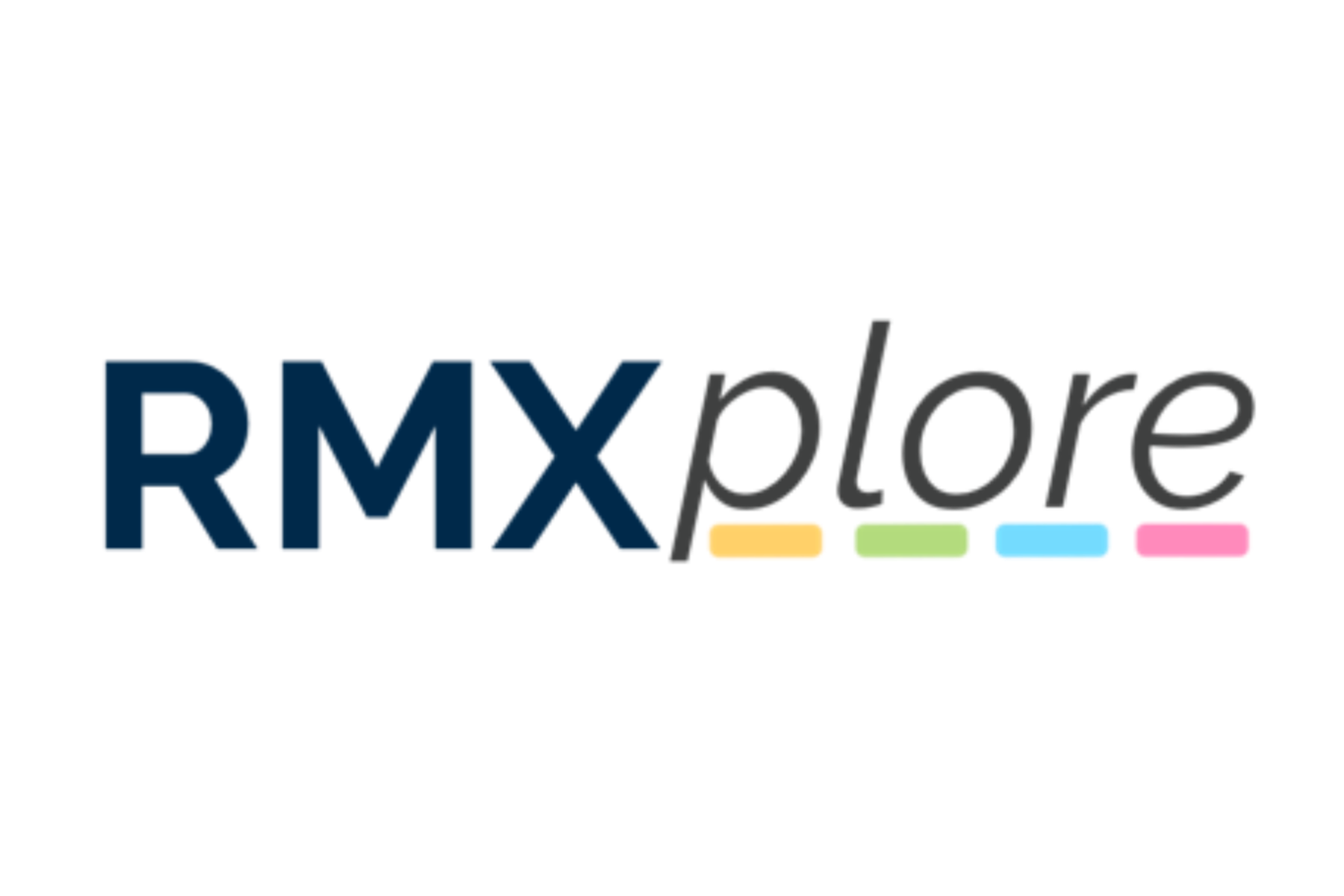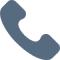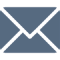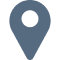# Operations, Measurement, and Thinking (OMT) III

Please note that RMXplore's Math Bytes Courses are not
currently open for registration
.

### Operations, Measurement, and Thinking (OMT) III

Coming Soon!
Course Pricing:
• Online : \$260
• Houston Center : \$320
• Cupertino Center : \$400
Course Logistics:
Duration : to be updated
Number of Classes: 5 weeks course
Occurs : to be updated

### Math Byte Course Topics:

Course Topics are organized as six modules with five granular topics covered under each module. Click through below to see all modules/topics for this course.

#### 01 - Additions and Subtraction• Ordering decimals and fractions
• Multiplication of decimals
• Multiplication of fractions
• Division of fractions

#### 02 - Multiplication and Division• Ordering decimals and fractions
• Multiplication of decimals
• Multiplication of fractions
• Division of fractions

#### 03 - Fractions• Clocks: adding in seconds and 24-hour military time
• Money: operating in decimal
• Area and perimeter of composite figures
• More on unit conversions
• Volume of a rectangular prism (liters and cm^3)

#### 04 - Measurement• Angle - notation and estimation
• Angles - straight line, point, vertically opposite, etc.
• Identify symmetric figures
• Complete symmetric figure
• Recognizing shapes that can tesselate,

#### 05 - Elementary Geometry• Creating tables from data
• Interpreting tables
• Creating line graphs
• Interpreting line graphs
• What is an average? finding total from average, etc.

#### 06 - Word Problems• Decimals and fractions
• 24-hour clocks and 4 operations of money
• Volume of a cube / rectangular prism
• Tables and line graphs
• Averages